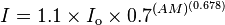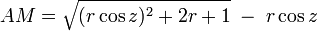We now have even more [and better presented] science information on our Science Issues page.

There we go into more detail wrt laser beam angles, air mass and scattering effects on Earth and Mars:

We do use some approximations and assumptions when calculating our laser impact upon Mars.

What we do know:

Laser specs: 3.5W and 1W blue lasers [<3mm initial beam diameter; divergence < 2.5 mRad (Full Angle), wavelength 450nm].

1W red (JetLaser) & 100mW red “Nano” laser [<4mm initial beam diameter; divergence <0.5mRad, wavelength 650nm].

We use approximations from the following equation [calculates sunlight intensity arriving at Earth’s surface], taking into account Air Mass and absorbtion/scattering, in order to calculate effect in laser light leaving the Earth’s surface. Our laser site is 100m above sea level so not significant enough to factor into the equation.Simplified Air Mass calculation:Mars: mean radius = 3389.5 Km

Earth: Mars distance varies from closest at opposition [55.7 Million Km] to furthest [401.3 Million Km]

We ignored the effects of the Mars atmosphere and averaged the effects of Rayleigh Scattering in Earth’s air mass.

We assumed accurate targetting of Mars, which not only requires line-of-sight accuracy using telescope, but also, when the earth-Mars light time exceeds 5 mins, requires slight adjustment (some anticipation within high-power field-of-view (FOV) to account for Mars progression over 10 minutes). In fact, we rarely targetted Mars when the light time exceeded 15mins.

We assumed an even spread of photons throughout the beam, which is rather inacurate. The centre of beam is more dense, hence if we are as accurate in our aim as we believe, there will be a hike in the true number of photons hitting Mars from our lasers.

Please see the ABOUT  section for a lot more on this and the results of our calculations.

We invite physicists to assess and criticise our assumptions and math: